# The Truncated Cube

The truncated cube is a 3D uniform polyhedron bounded by 14 polygons (8 triangles and 6 octagons), 36 edges, and 24 vertices. It may be constructed by truncating the cube's vertices at (2-√2) of its edge length. More simply, it may be constructed by radially expanding the cube's edges outwards by 1/√2 of its edge length and taking the convex hull. It may also be constructed by radially expanding the triangular faces of the cuboctahedron outwards.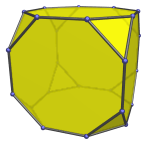The truncated cube can be augmented with a square cupola (J4) to produce an augmented truncated cube (J66), one of the Johnson solids. Adding a second square cupola on the opposite side produces the biaugmented truncated cube (J67).

## Projections

In order to be able to identify the truncated cube in various projections of 4D objects, it is useful to know how it appears from various viewpoints. The following are some of the commonly-encountered views:

Projection Envelope Description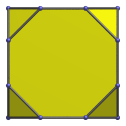Square

Parallel projection centered on an octagonal face.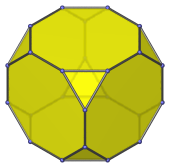Regular dodecagon

Parallel projection centered on a triangular face.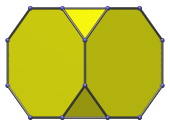Octagon

Parallel projection centered on an edge shared between two octagons.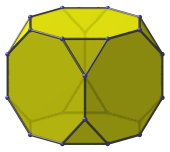Dodecagon

Vertex-centered parallel projection.

## Coordinates

The Cartesian coordinates of the truncated cube, centered on the origin and having edge length 2, are all permutations of coordinates and changes of sign of:

• (1, (1+√2), (1+√2))

## Occurrences

The truncated cube occurs as cells in the following uniform polychora:

It also occurs in the following CRF polychora (amongst others):

Last updated 17 Jun 2019.